Next: Planetary Rotation Up: Terrestrial Ocean Tides Previous: Expansion of Tide Generating

# Surface Harmonics and Solid Harmonics

A surface harmonic of degree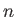(whereis a non-negative integer), denoted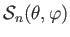, is defined as a well-behaved solution to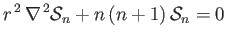(12.36)

on the surface of a sphere (i.e.,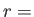constant). Here,,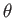,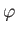are standard spherical coordinates, and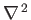is the Laplacian operator. It follows that (Love 1927)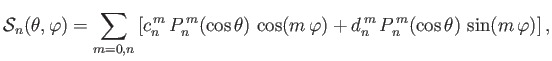(12.37)

where the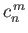and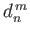are arbitrary coefficients, and the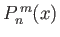associated Legendre functions.

A solid harmonic of degree(whereis a non-negative integer), denoted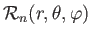, is defined as a well-behaved solution to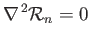(12.38)

in the interior of a sphere (i.e., the region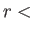constant). It follows that (Love 1927)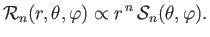(12.39)

In particular, the functions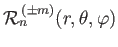and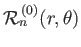, introduced in Section 12.3, are solid harmonics of degree. Note that the Cartesian coordinates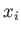(where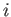runs from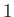to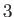) are solid harmonics of degree. Moreover,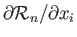is a solid harmonic of degree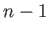. Finally,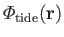, specified in Equation (12.35), is a solid harmonic of degree 2.

The following results regarding solid harmonics are useful (Love 1927):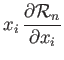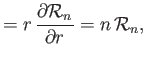(12.40)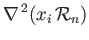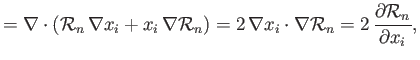(12.41)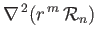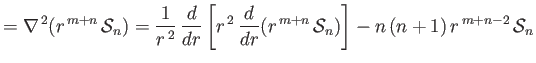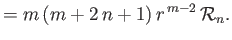(12.42)

Here, use has been made of the Einstein summation convention (Riley 1974).Next: Planetary Rotation Up: Terrestrial Ocean Tides Previous: Expansion of Tide Generating
Richard Fitzpatrick 2016-01-22